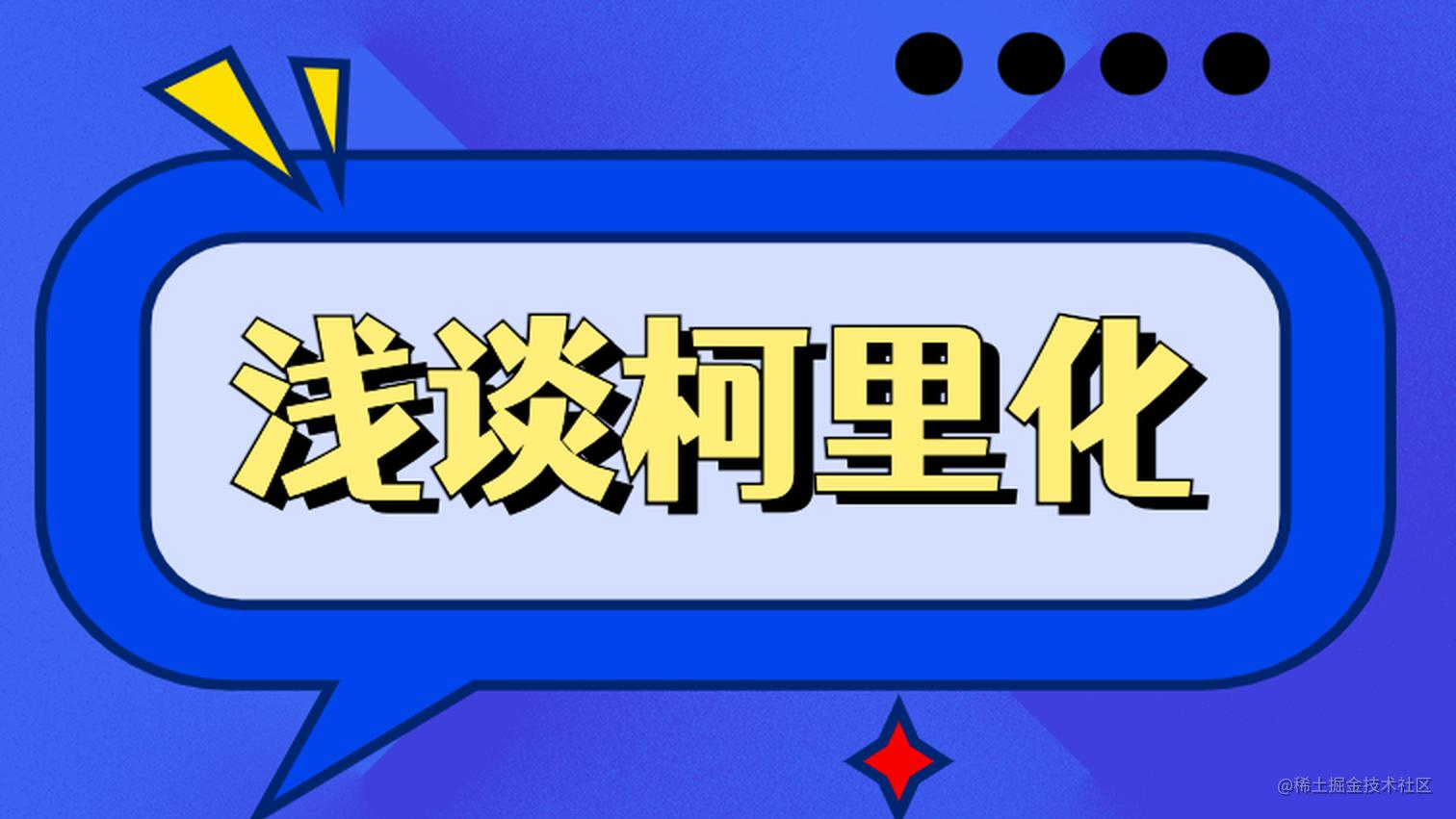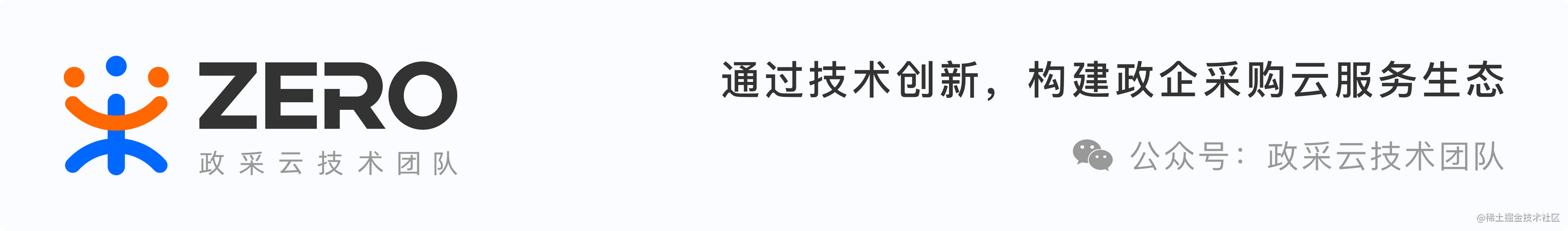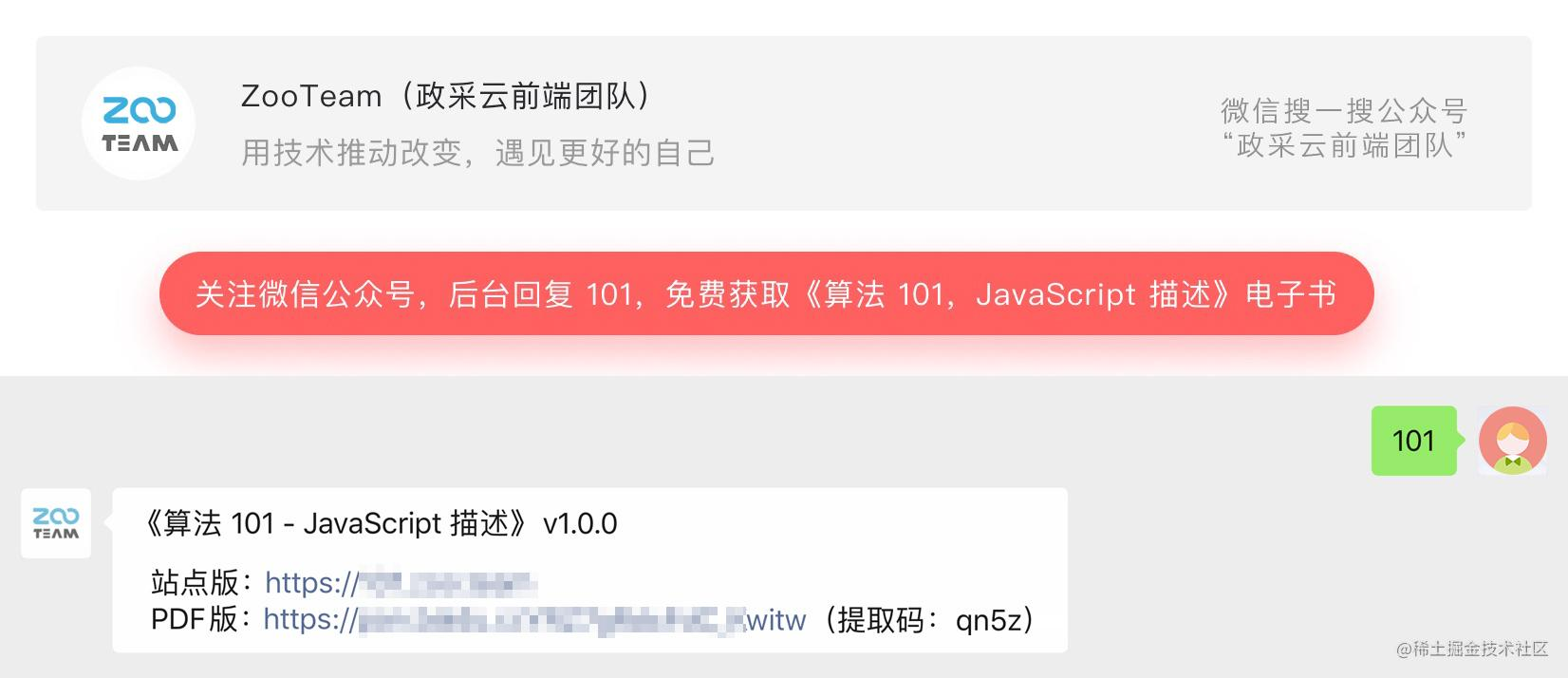# 浅谈柯里化##### 背景：

``````import React from "react";
​
const accountReg = /^[a-zA-Z0-9_-]{4,16}\$/;
​
const FormCom = () => {
​
const checkReg = (reg, txt) => {
return reg.test(txt)
}

//账号
const checkAccount = (event) => {
checkReg(accountReg, event.target.value);
// 其他逻辑
};
​
//密码
const checkPassword = (event) => {
// 其他逻辑
};

...
// 省去其他函数校验

render() {
return (
<form>
账号：
<input onChange={checkAccount} type="text" name="account" />
密码：
</form>
);
}
}
​
export default FormCom;

``````import React from "react";
​
const accountReg = /^[a-zA-Z0-9_-]{4,16}\$/;
​
const FormCom = () => {

// 柯里化封装
const curryCheck = (reg) => {
return function(txt) {
return reg.test(txt)
}
}
​
//账号，这样就省去了一个参数的传递
const checkAccount = curryCheck(accountReg);
​
//密码，这样就省去了一个参数的传递
​
const checkAccountFn = () => {
checkAccount(event.target.value);
// 其他逻辑
}

const passwordFn = (event) => {
// 其他逻辑
};

...
// 省去其他函数校验
​
render() {
return (
<form>
账号：
<input onChange={checkAccountFn} type="text" name="account" />
密码：
</form>
);
}
}
​
export default FormCom;

##### 一、柯里化

``````  const addFn = (x, y, z) => {
return x + y + z;
};
​
​
// 将上述过程转化为下面的实现过程就是柯里化
​
const sumFn = (x) => {
return function(y) {
return function(z) {
return x + y + z;
}
}
}
​
const sum = sumFn(1)(2)(3);

``````const simplifySumFn = x => y => z => {
return x + y + z;
}

1. 单一原则：在函数式编程中，往往是让一个函数处理的问题尽可能单一，而不是一个函数处理多个任务。
2. 提高维护性以及降低代码的重复性
##### 二、柯里化的场景

1、比如我们在求和中，以一定的数字为基数进行累加的时候，就用到了函数柯里化。当然函数柯里化感觉上是把简答的问题复杂化了，其实不然。比如：

``````// 比如，基础分值是30 + 30；
const fractionFn = (x) => {
const totalFraction =  x + x;
return function(num) {
}
};
​
const baseFn = fractionFn(30);
const base1Fn = baseFn(1);
const base2Fn = baseFn(2);

2、上述也可是实现打印日志的功能函数，细心的你不知道你发现了没，其实date, type每次还需要传参。是不是可以进行抽离呢，当然了，函数柯里化就可以完美的解决这个。

``````const date = new Date();
​
const logFn = (date, type, msg) => {
console.log(`\${date.getHours()} : \${date.getMinutes()} \${type} - \${msg}`);
}
​
logFn(date, 'warning', '声明的变量未使用');
logFn(date, 'warning', '暂未查询到数据');

``````const date = new Date();
const logFn = date => type => msg => {
console.log(`\${date.getHours()}:\${date.getMinutes()} \${type} - \${msg}`);
}
const nowLogFn = logFn(date);
nowLogFn('warning')('声明的变量未被引用');

##### 三、柯里化函数的实现

``````const selfCurryFn = (fn) => {
const fnLen = fn.length;  // fn 接收的参数
function curry(...args) {
const argLen = args.length; // curry 接收的参数
if(argLen >= fnLen) {
return fn.apply(this, args); // 如果外面绑定 this 的话，直接绑定到fn上
} else {
// 参数个数没有达到时继续接收剩余的参数
function otherCurry(...args2) {
return curry.apply(this, args.concat(args2))
}
return otherCurry;
}
}
return curry;
}
•
const selfAddFn = (x, y, z) => {
return x + y + z;
}
•
•
console.log("🚀 ~ file: preview.html ~ line 80 ~ selfSum(1, 2, 3)", selfSum(1, 2, 3))
console.log("🚀 ~ file: preview.html ~ line 81 ~ selfSum(1, 2)(3)", selfSum(1, 2)(3))
console.log("🚀 ~ file: preview.html ~ line 81 ~ selfSum(1, 2)(3)", selfSum(1)(2)(3))

##### 四、柯里化在其他库的应用

1、我们常用的 Redux，里面其实也用到了柯里化。Redux 的中间件提供的是位于 action 被发起之后数据流加上中间件后变成了

view -> action -> middleware -> reducer -> store 。在 middleware 这个节点可以进行一些“副作用”的 操作，比如打印日志等等。

``````export default function applyMiddleware(...middlewares) {
return createStore => (...args) => {
// 利用传入的 createStore 和 reducer 和创建一个 store
const store = createStore(...args)
let dispatch = () => {
throw new Error()
}
const middlewareAPI = {
getState: store.getState,
dispatch: (...args) => dispatch(...args)
}
// middlewareAPI 这个参数分别执行一遍
const chain = middlewares.map(middleware => middleware(middlewareAPI))
// 组装成一个新的函数，即新的 dispatch
dispatch = compose(...chain)(store.dispatch)
return {
...store,
dispatch
}
}
}

2、组合函数是 JavaScript 开发过程中一种对函数的使用组合模式。

• 我们对某一个函数进行调用，执行 fn1、fn2，这两个函数是依次执行
• 每次我们都需要进行两个函数的调用，操作上就会显示的重复
• 那么我们是不是可以将 fn1、fn2 组合起来，自动一次调用呢？ 其实实现上述的过程就是组合函数（compose function）。
``````const fn1 = (num) => {
return num + 10;
}
​
const fn2 = (num) => {
return num * num;
}
​
const a = 10;
const result = fn2(fn1(a));

``````function compose (fn1, fn2) {
return function(num) {
return fn2(fn1(num));
}
}
​
const fn1 = (num) => {
return num + 10;
}
​
const fn2 = (num) => {
return num * num;
}
​
const a = 10;
const newFn = compose(fn1, fn2);
const result = newFn(a);

``````function createCompose(...fns) {
const length = fns.length;
for(let i = 0; i < length; i++) {
if(typeof fns[i] !== 'function') {
throw new TypeError('arguments is not function');
}
}

function compose(...args ) {
let index = 0;
let result = length ? fns[index].apply(this, args) : args;
while(++index < length) {
result = fns[index].call(this, result);
}
}
return compose;
}

##### 总结：
1. 柯里化可以让我们给一个函数传递较少的参数得到一个记住某些固定参数的新函数
2. 这是对函数的一种“缓存”
3. 使函数变得更加灵活、颗粒度更小
4. 可以把多元函数转换成一元函数，可以组合使用函数产生更强的功能
##### 参考链接：

JavaScript专题之函数柯里化

## 推荐阅读

Rollup 与 Webpack 的 Tree-shaking

Git 是如何工作的

• 政采云前端小报

• 商品选择 sku 插件

## 招贤纳士